Скачать презентацию The Basics of X-Ray Timing Gian Luca Israel

• Количество слайдов: 38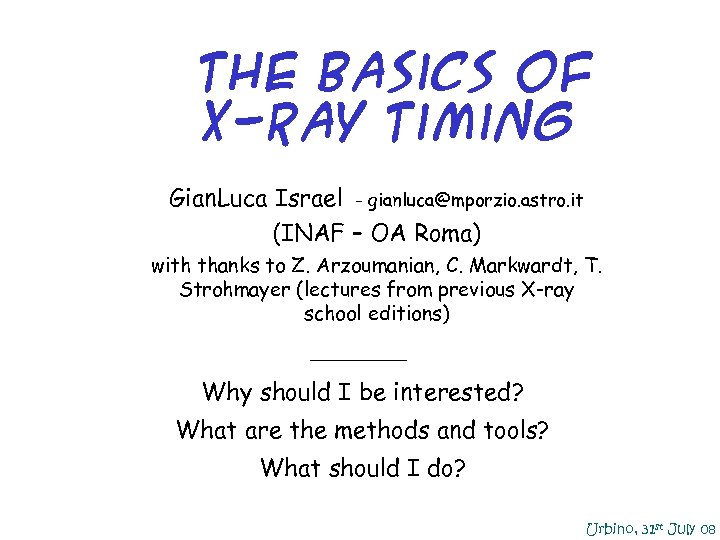The Basics of X-Ray Timing Gian. Luca Israel - [email protected] astro. it (INAF – OA Roma) with thanks to Z. Arzoumanian, C. Markwardt, T. Strohmayer (lectures from previous X-ray school editions) • Why should I be interested? • What are the methods and tools? • What should I do? Urbino, 31 st July 08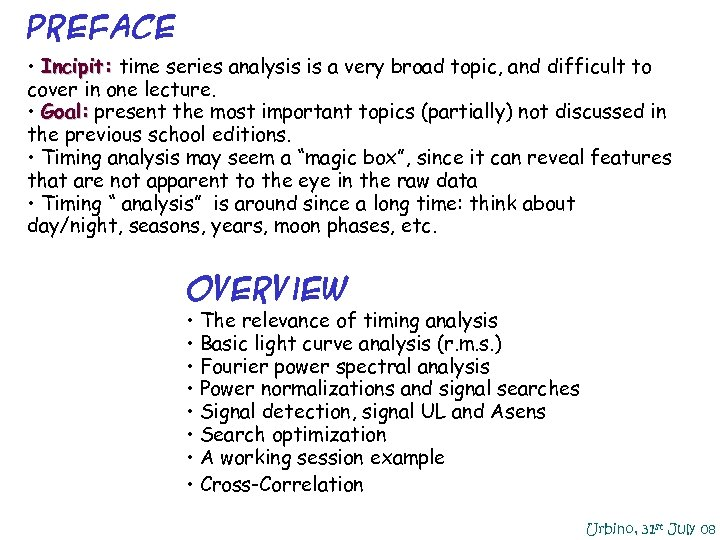Preface • Incipit: time series analysis is a very broad topic, and difficult to cover in one lecture. • Goal: present the most important topics (partially) not discussed in the previous school editions. • Timing analysis may seem a “magic box”, since it can reveal features that are not apparent to the eye in the raw data • Timing “ analysis” is around since a long time: think about day/night, seasons, years, moon phases, etc. Overview • The relevance of timing analysis • Basic light curve analysis (r. m. s. ) • Fourier power spectral analysis • Power normalizations and signal searches • Signal detection, signal UL and Asens • Search optimization • A working session example • Cross-Correlation Urbino, 31 st July 08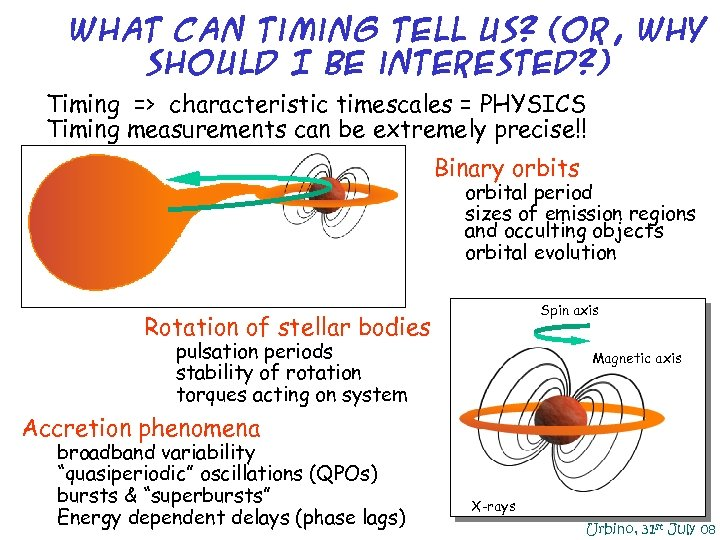What can Timing Tell Us? (or, why should I be interested? ) • Timing => characteristic timescales = PHYSICS • Timing measurements can be extremely precise!! • Binary orbits – orbital period – sizes of emission regions and occulting objects – orbital evolution Spin axis • Rotation of stellar bodies – pulsation periods – stability of rotation – torques acting on system Magnetic axis Accretion phenomena broadband variability “quasiperiodic” oscillations (QPOs) bursts & “superbursts” Energy dependent delays (phase lags) X-rays Urbino, 31 st July 08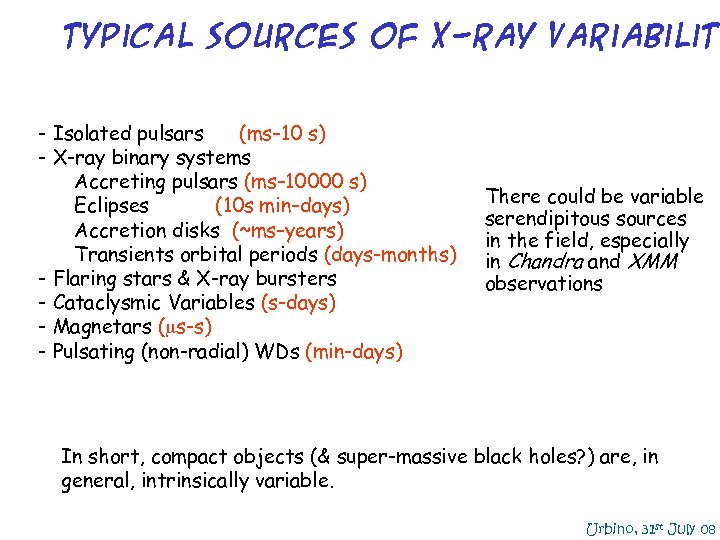Typical Sources of X-Ray Variability - Isolated pulsars (ms– 10 s) - X-ray binary systems Accreting pulsars (ms– 10000 s) • Eclipses (10 s min–days) Accretion disks (~ms–years) Transients orbital periods (days-months) - Flaring stars & X-ray bursters - Cataclysmic Variables (s-days) - Magnetars (ms-s) - Pulsating (non-radial) WDs (min-days) There could be variable serendipitous sources in the field, especially in Chandra and XMM observations In short, compact objects (& super-massive black holes? ) are, in general, intrinsically variable. Urbino, 31 st July 08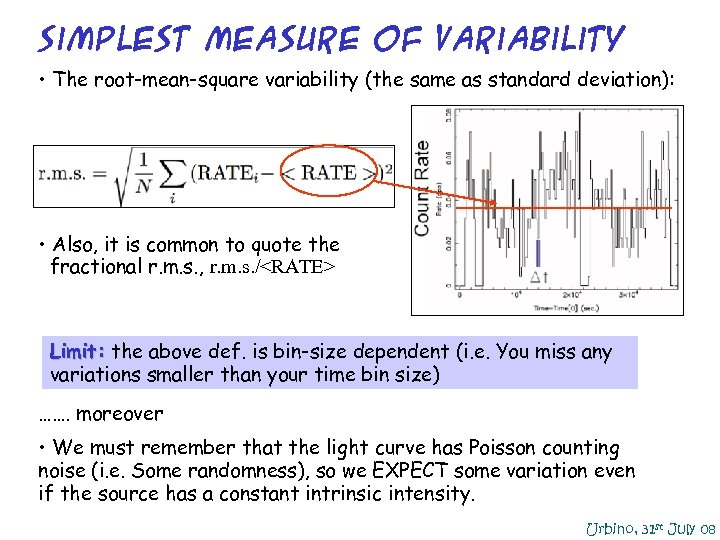Simplest Measure of Variability • The root-mean-square variability (the same as standard deviation): • Also, it is common to quote the fractional r. m. s. , r. m. s. / Limit: the above def. is bin-size dependent (i. e. You miss any variations smaller than your time bin size) ……. moreover • We must remember that the light curve has Poisson counting noise (i. e. Some randomness), so we EXPECT some variation even if the source has a constant intrinsic intensity. Urbino, 31 st July 08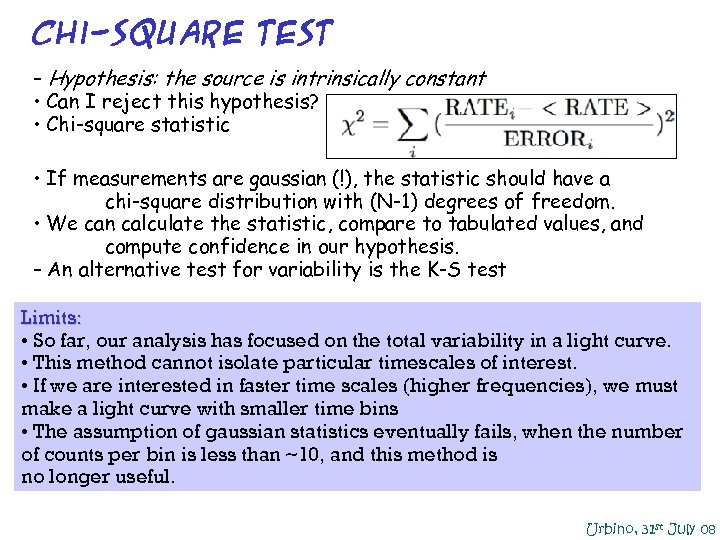Chi-Square Test – Hypothesis: the source is intrinsically constant • Can I reject this hypothesis? • Chi-square statistic • If measurements are gaussian (!), the statistic should have a chi-square distribution with (N-1) degrees of freedom. • We can calculate the statistic, compare to tabulated values, and compute confidence in our hypothesis. – An alternative test for variability is the K-S test Limits: • So far, our analysis has focused on the total variability in a light curve. • This method cannot isolate particular timescales of interest. • If we are interested in faster time scales (higher frequencies), we must make a light curve with smaller time bins • The assumption of gaussian statistics eventually fails, when the number of counts per bin is less than ~10, and this method is no longer useful. Urbino, 31 st July 08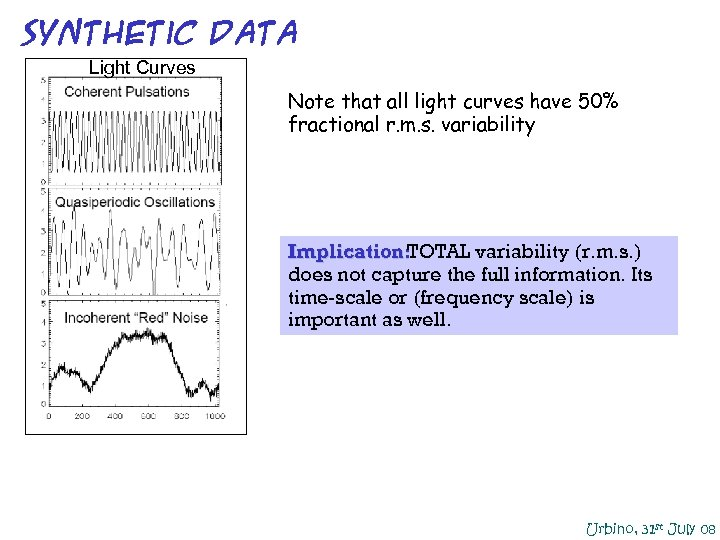Synthetic DATA Light Curves Note that all light curves have 50% fractional r. m. s. variability Implication: TOTAL variability (r. m. s. ) does not capture the full information. Its time-scale or (frequency scale) is important as well. Urbino, 31 st July 08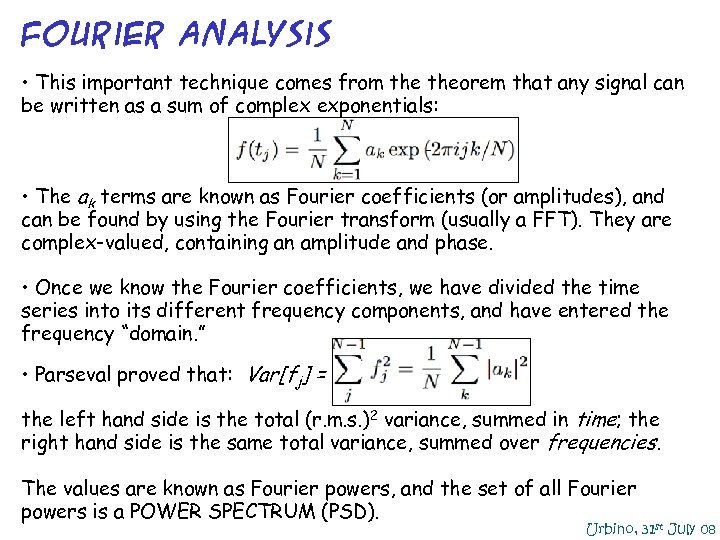Fourier Analysis • This important technique comes from theorem that any signal can be written as a sum of complex exponentials: • The ak terms are known as Fourier coefficients (or amplitudes), and can be found by using the Fourier transform (usually a FFT). They are complex-valued, containing an amplitude and phase. • Once we know the Fourier coefficients, we have divided the time series into its different frequency components, and have entered the frequency “domain. ” • Parseval proved that: Var[fj] = the left hand side is the total (r. m. s. )2 variance, summed in time; the right hand side is the same total variance, summed over frequencies. The values are known as Fourier powers, and the set of all Fourier powers is a POWER SPECTRUM (PSD). Urbino, 31 st July 08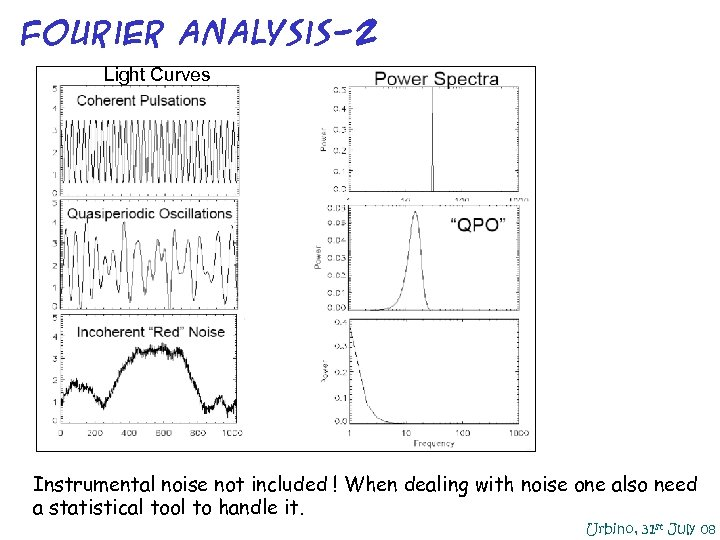Fourier Analysis-2 Light Curves Instrumental noise not included ! When dealing with noise one also need a statistical tool to handle it. Urbino, 31 st July 08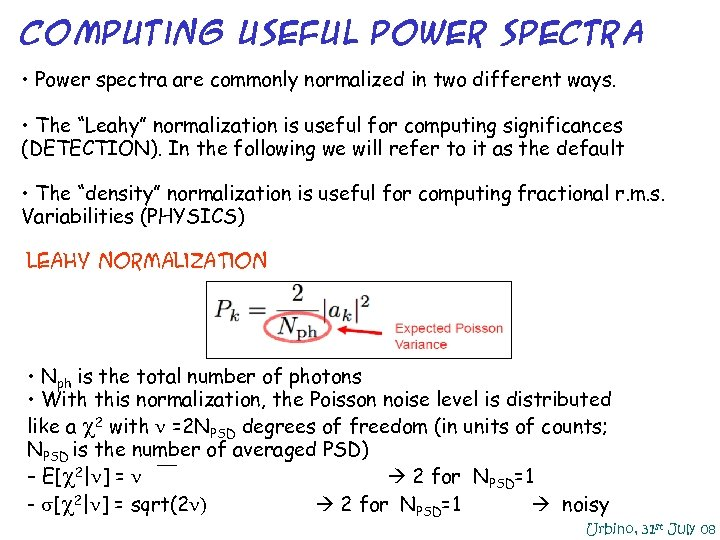Computing Useful Power Spectra • Power spectra are commonly normalized in two different ways. • The “Leahy” normalization is useful for computing significances (DETECTION). In the following we will refer to it as the default • The “density” normalization is useful for computing fractional r. m. s. Variabilities (PHYSICS) Leahy Normalization • Nph is the total number of photons • With this normalization, the Poisson noise level is distributed like a 2 with =2 NPSD degrees of freedom (in units of counts; NPSD is the number of averaged PSD) – E[ 2| ] = `` 2 for NPSD=1 - [ 2| ] = sqrt(2 ) 2 for NPSD=1 noisy Urbino, 31 st July 08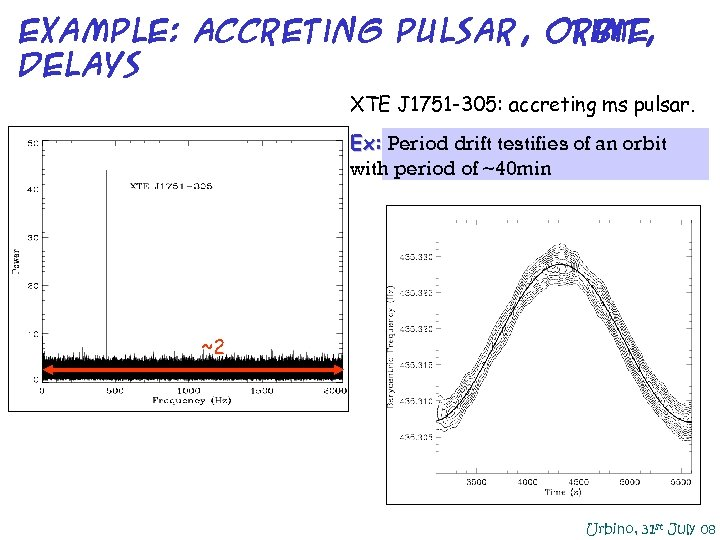Example: Accreting Pulsar, orbit, ti. ME delays XTE J 1751 -305: accreting ms pulsar. Ex: Period drift testifies of an orbit with period of ~40 min ~2 Urbino, 31 st July 08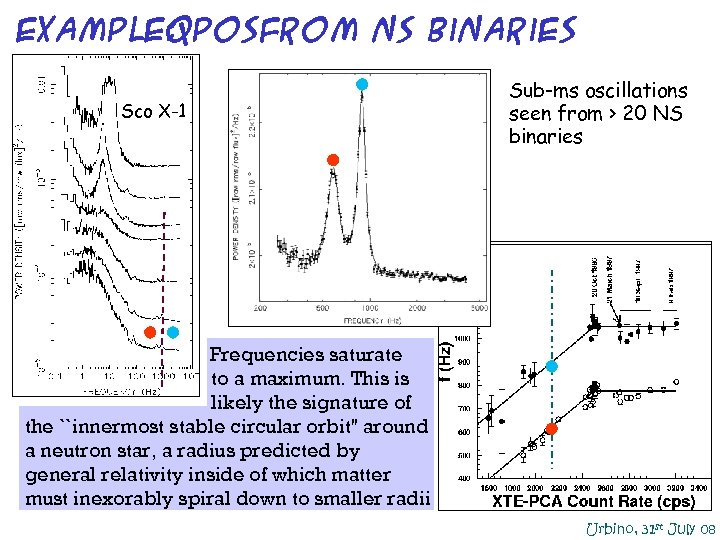Example: QPOsfrom NS Binaries Sco X-1 • Sub-ms oscillations seen from > 20 NS binaries Frequencies saturate to a maximum. This is likely the signature of the ``innermost stable circular orbit'' around a neutron star, a radius predicted by general relativity inside of which matter must inexorably spiral down to smaller radii Urbino, 31 st July 08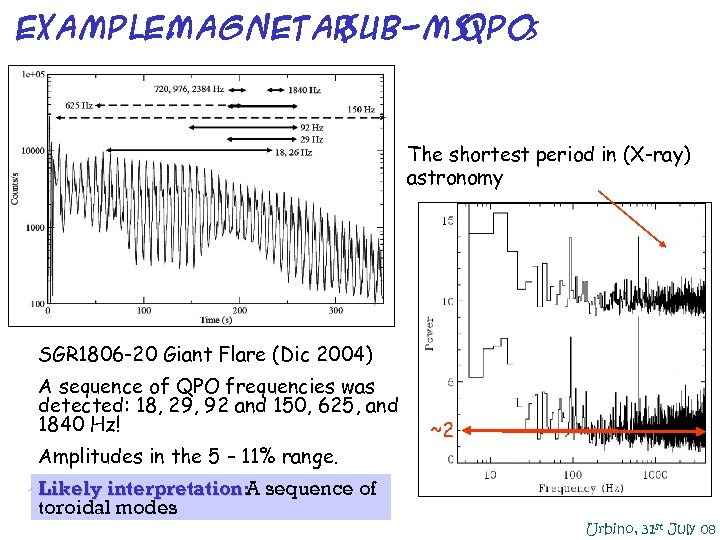Example: Magnetar sub-ms QPO s The shortest period in (X-ray) astronomy • SGR 1806 -20 Giant Flare (Dic 2004) • A sequence of QPO frequencies was detected: 18, 29, 92 and 150, 625, and 1840 Hz! • Amplitudes in the 5 – 11% range. ~2 • Likely interpretation: sequence of A toroidal modes Urbino, 31 st July 08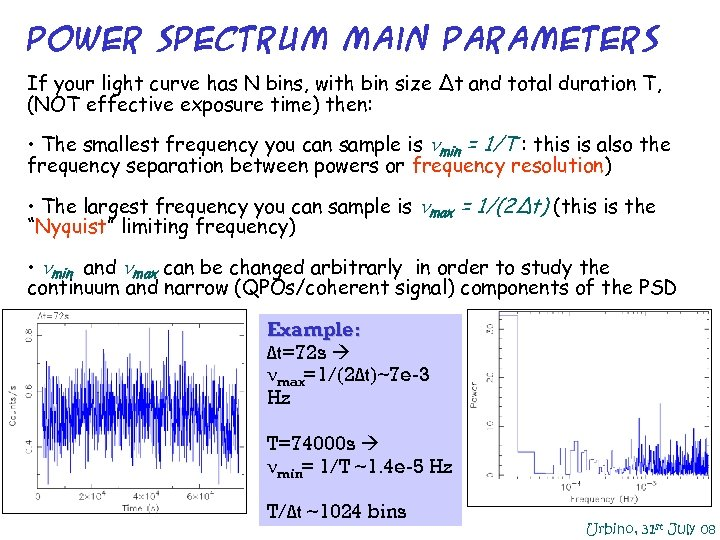Power Spectrum main parameters If your light curve has N bins, with bin size Δt and total duration T, (NOT effective exposure time) then: • The smallest frequency you can sample is nmin = 1/T : this is also the frequency separation between powers or frequency resolution) • The largest frequency you can sample is nmax = 1/(2Δt) (this is the “Nyquist” limiting frequency) • nmin and nmax can be changed arbitrarly in order to study the continuum and narrow (QPOs/coherent signal) components of the PSD Example: Δt=72 s max=1/(2Δt)~7 e-3 Hz T=74000 s min= 1/T ~1. 4 e-5 Hz T/Δt ~1024 bins Urbino, 31 st July 08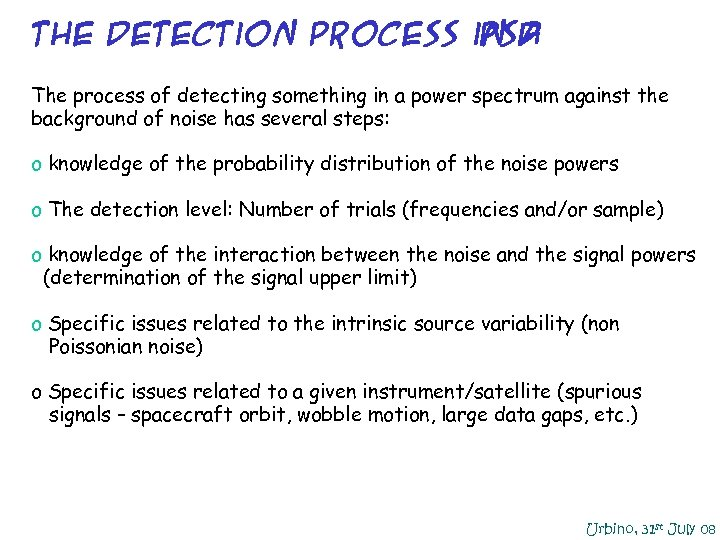The detection process in a psd The process of detecting something in a power spectrum against the background of noise has several steps: o knowledge of the probability distribution of the noise powers o The detection level: Number of trials (frequencies and/or sample) o knowledge of the interaction between the noise and the signal powers (determination of the signal upper limit) o Specific issues related to the intrinsic source variability (non Poissonian noise) o Specific issues related to a given instrument/satellite (spurious signals – spacecraft orbit, wobble motion, large data gaps, etc. ) Urbino, 31 st July 08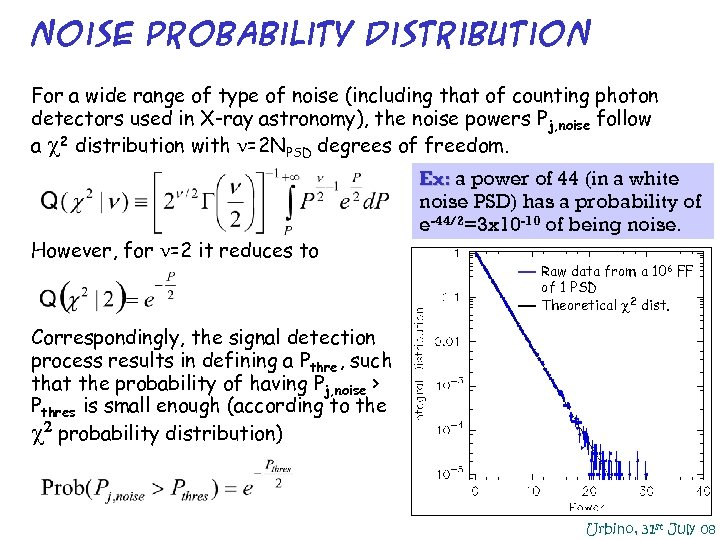Noise probability distribution For a wide range of type of noise (including that of counting photon detectors used in X-ray astronomy), the noise powers Pj, noise follow a 2 distribution with =2 NPSD degrees of freedom. Ex: a power of 44 (in a white noise PSD) has a probability of e-44/2=3 x 10 -10 of being noise. However, for =2 it reduces to Raw data from a 106 FF of 1 PSD Theoretical 2 dist. Correspondingly, the signal detection process results in defining a Pthre , such that the probability of having Pj, noise > Pthres is small enough (according to the 2 probability distribution) Urbino, 31 st July 08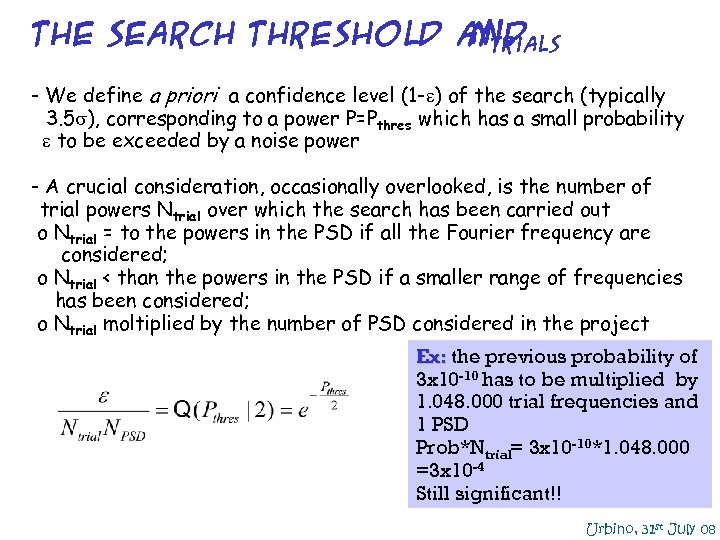the search threshold and Ntrials - We define a priori a confidence level (1 -e) of the search (typically - 3. 5 ), corresponding to a power P=Pthres which has a small probability -e to be exceeded by a noise power - A crucial consideration, occasionally overlooked, is the number of -trial powers Ntrial over which the search has been carried out o Ntrial = to the powers in the PSD if all the Fourier frequency are considered; o Ntrial < than the powers in the PSD if a smaller range of frequencies has been considered; o Ntrial moltiplied by the number of PSD considered in the project Ex: the previous probability of 3 x 10 -10 has to be multiplied by 1. 048. 000 trial frequencies and 1 PSD Prob*Ntrial= 3 x 10 -10*1. 048. 000 =3 x 10 -4 Still significant!! Urbino, 31 st July 08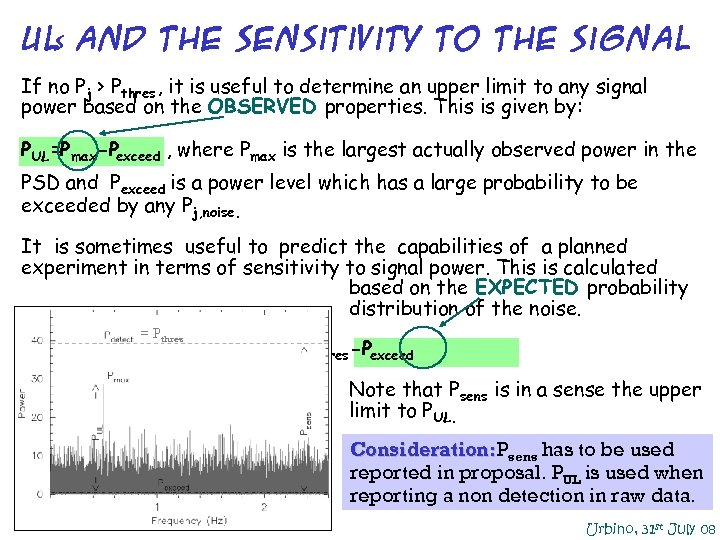UL and THE sensitivity to the signal s If no Pj > Pthres , it is useful to determine an upper limit to any signal power based on the OBSERVED properties. This is given by: PUL=Pmax-Pexceed , where Pmax is the largest actually observed power in the PSD and Pexceed is a power level which has a large probability to be exceeded by any Pj, noise. It is sometimes useful to predict the capabilities of a planned experiment in terms of sensitivity to signal power. This is calculated based on the EXPECTED probability distribution of the noise. = Pthres Psens =Pthres -Pexceed Note that Psens is in a sense the upper limit to P UL. Consideration: Psens has to be used reported in proposal. PUL is used when reporting a non detection in raw data. Urbino, 31 st July 08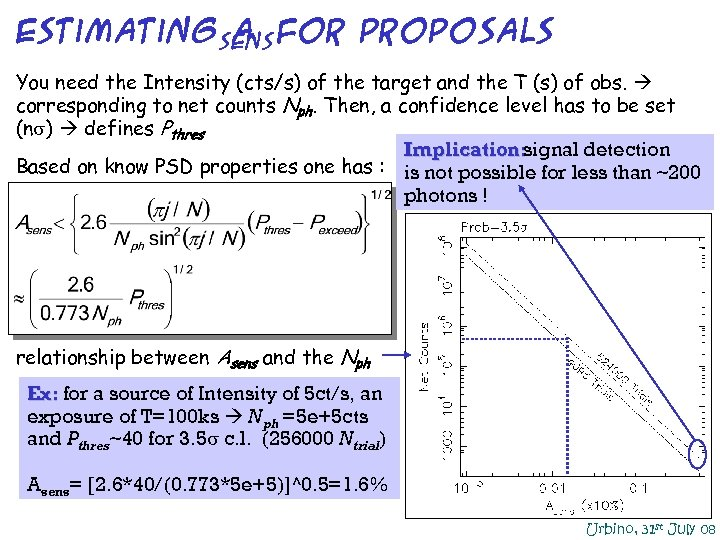Estimatingsens for Proposals A You need the Intensity (cts/s) of the target and the T (s) of obs. corresponding to net counts Nph. Then, a confidence level has to be set (n ) defines Pthres Implication: signal detection Based on know PSD properties one has : is not possible for less than ~200 photons ! relationship between Asens and the Nph Ex: for a source of Intensity of 5 ct/s, an exposure of T=100 ks Nph =5 e+5 cts and P thres~40 for 3. 5 c. l. (256000 Ntrial) Asens= [2. 6*40/(0. 773*5 e+5)]^0. 5=1. 6% Urbino, 31 st July 08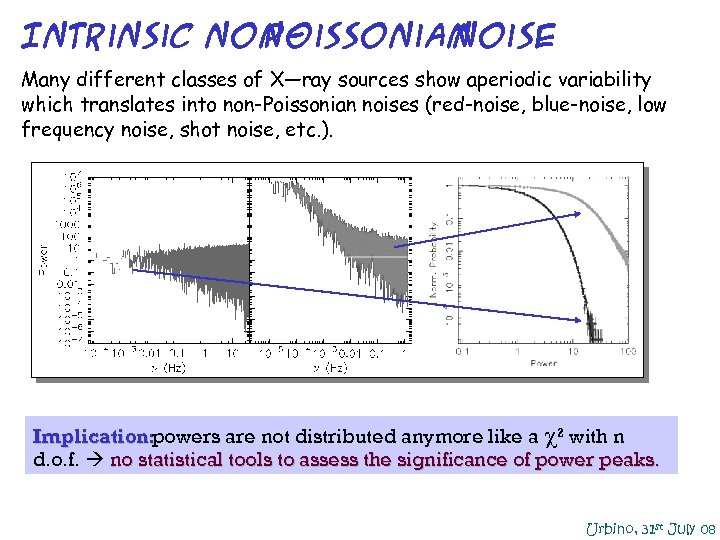Intrinsic non. Poissonian noise Many different classes of X—ray sources show aperiodic variability which translates into non-Poissonian noises (red-noise, blue-noise, low frequency noise, shot noise, etc. ). Implication: powers are not distributed anymore like a 2 with n d. o. f. no statistical tools to assess the significance of power peaks. Urbino, 31 st July 08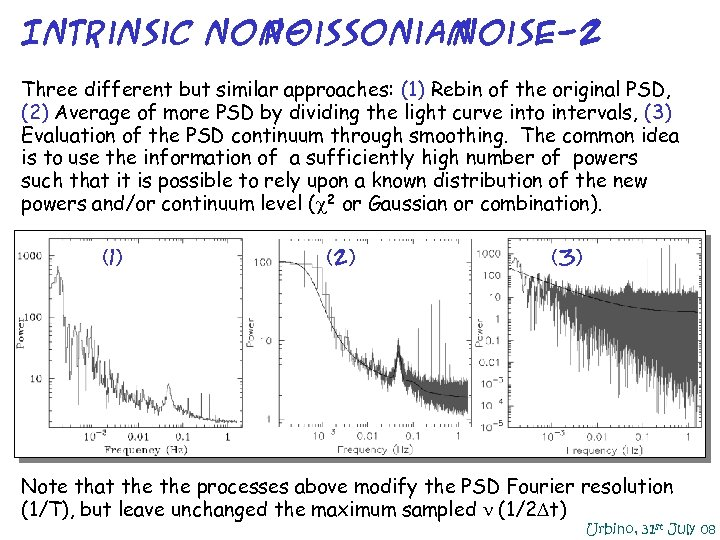Intrinsic non. Poissonian noise-2 Three different but similar approaches: (1) Rebin of the original PSD, (2) Average of more PSD by dividing the light curve into intervals, (3) Evaluation of the PSD continuum through smoothing. The common idea is to use the information of a sufficiently high number of powers such that it is possible to rely upon a known distribution of the new powers and/or continuum level ( 2 or Gaussian or combination). (1) (2) (3) Note that the processes above modify the PSD Fourier resolution (1/T), but leave unchanged the maximum sampled (1/2 Dt) Urbino, 31 st July 08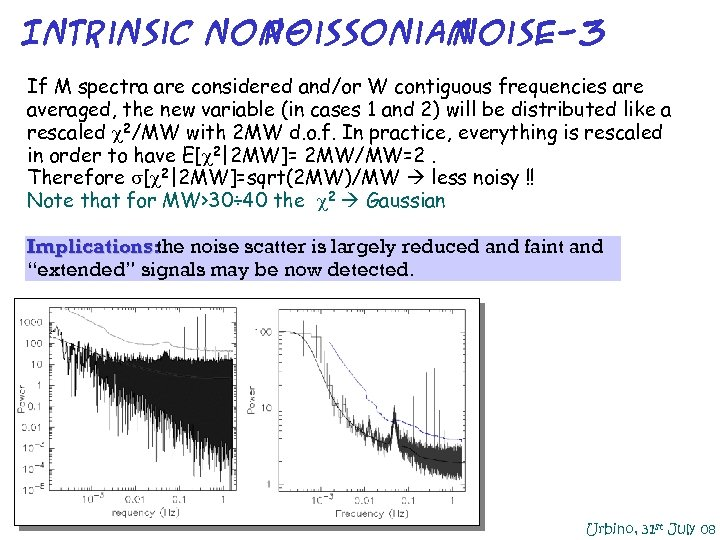Intrinsic non. Poissonian noise-3 If M spectra are considered and/or W contiguous frequencies are averaged, the new variable (in cases 1 and 2) will be distributed like a rescaled 2/MW with 2 MW d. o. f. In practice, everything is rescaled in order to have E[ 2|2 MW]= 2 MW/MW=2. Therefore [ 2|2 MW]=sqrt(2 MW)/MW less noisy !! Note that for MW>30÷ 40 the 2 Gaussian Implications: noise scatter is largely reduced and faint and the “extended” signals may be now detected. Urbino, 31 st July 08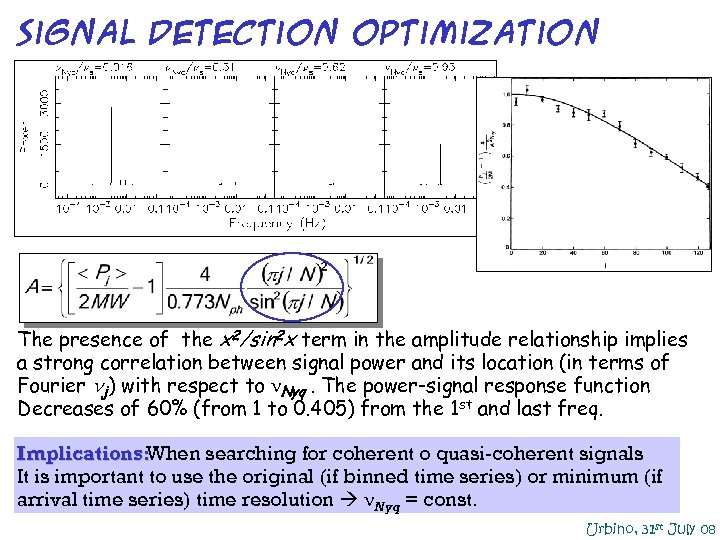signal detection optimization The presence of the x 2/sin 2 x term in the amplitude relationship implies a strong correlation between signal power and its location (in terms of Fourier nj) with respect to Nyq. The power-signal response function Decreases of 60% (from 1 to 0. 405) from the 1 st and last freq. Implications: When searching for coherent o quasi-coherent signals It is important to use the original (if binned time series) or minimum (if arrival time series) time resolution Nyq = const. Urbino, 31 st July 08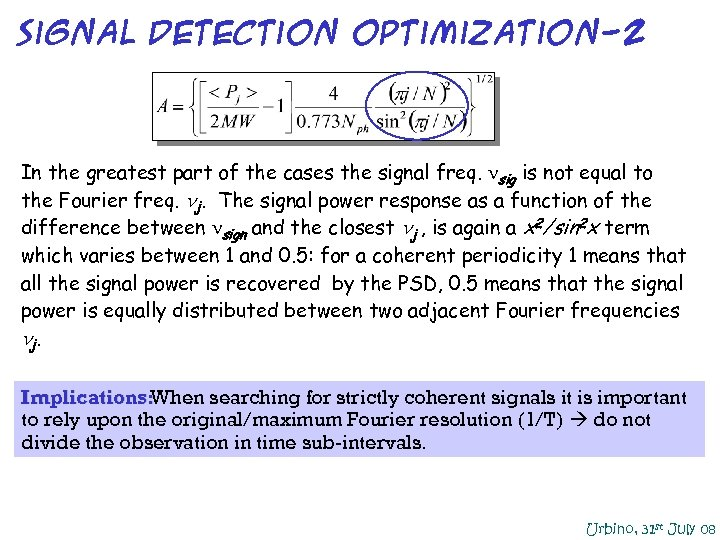signal detection optimization-2 In the greatest part of the cases the signal freq. sig is not equal to the Fourier freq. nj. The signal power response as a function of the difference between sign and the closest nj , is again a x 2/sin 2 x term which varies between 1 and 0. 5: for a coherent periodicity 1 means that all the signal power is recovered by the PSD, 0. 5 means that the signal power is equally distributed between two adjacent Fourier frequencies nj. Implications: When searching for strictly coherent signals it is important to rely upon the original/maximum Fourier resolution (1/T) do not divide the observation in time sub-intervals. Urbino, 31 st July 08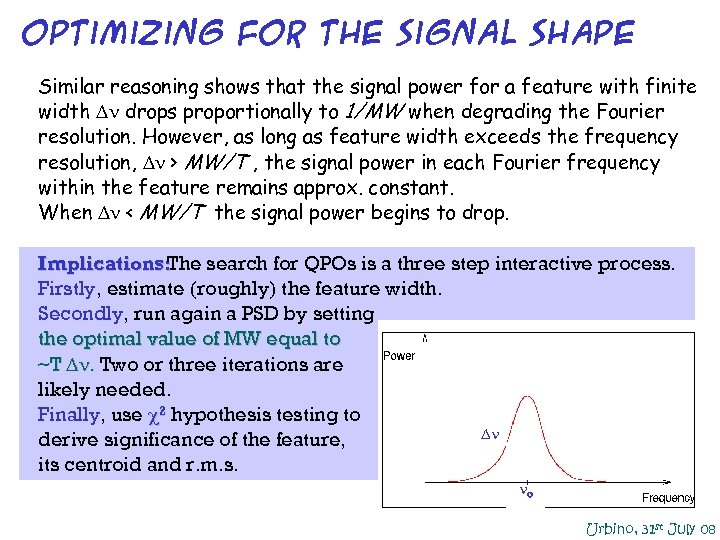optimizing for the signal shape Similar reasoning shows that the signal power for a feature with finite width D drops proportionally to 1/MW when degrading the Fourier resolution. However, as long as feature width exceeds the frequency resolution, D > MW/T , the signal power in each Fourier frequency within the feature remains approx. constant. When D < MW/T the signal power begins to drop. Implications: The search for QPOs is a three step interactive process. Firstly, estimate (roughly) the feature width. Secondly, run again a PSD by setting the optimal value of MW equal to ~T D. Two or three iterations are likely needed. Finally, use 2 hypothesis testing to D derive significance of the feature, its centroid and r. m. s. o Urbino, 31 st July 08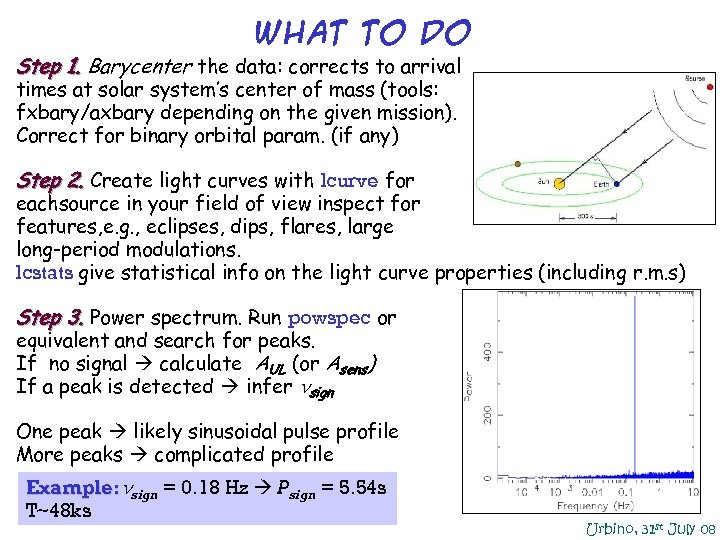What to do Step 1. Barycenter the data: corrects to arrival times at solar system’s center of mass (tools: fxbary/axbary depending on the given mission). Correct for binary orbital param. (if any) Step 2. Create light curves with lcurve for eachsource in your field of view inspect for features, e. g. , eclipses, dips, flares, large long-period modulations. lcstats give statistical info on the light curve properties (including r. m. s) Step 3. Power spectrum. Run powspec or equivalent and search for peaks. If no signal calculate AUL (or Asens) If a peak is detected infer nsign One peak likely sinusoidal pulse profile More peaks complicated profile Example: nsign = 0. 18 Hz P sign = 5. 54 s T~48 ks Urbino, 31 st July 08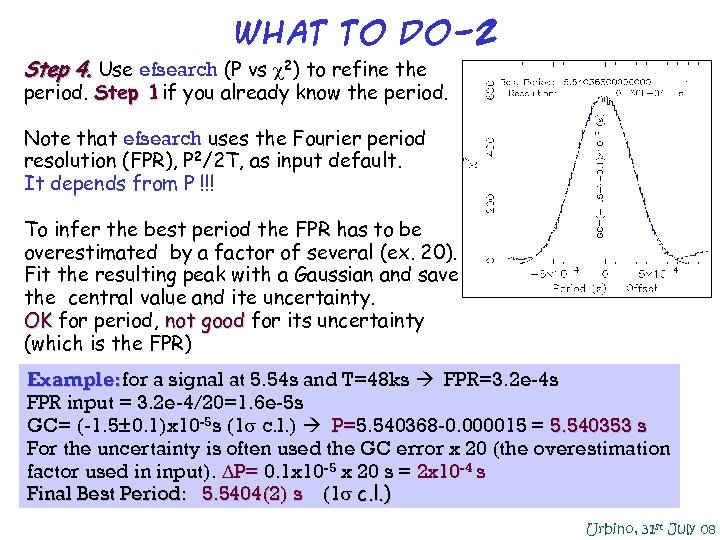What to do-2 Step 4. Use efsearch (P vs 2) to refine the period. Step 1 if you already know the period. Note that efsearch uses the Fourier period resolution (FPR), P 2/2 T, as input default. It depends from P !!! To infer the best period the FPR has to be overestimated by a factor of several (ex. 20). Fit the resulting peak with a Gaussian and save the central value and ite uncertainty. OK for period, not good for its uncertainty (which is the FPR) Example: for a signal at 5. 54 s and T=48 ks FPR=3. 2 e-4 s FPR input = 3. 2 e-4/20=1. 6 e-5 s GC= (-1. 5± 0. 1)x 10 -5 s (1 c. l. ) P=5. 540368 -0. 000015 = 5. 540353 s P= For the uncertainty is often used the GC error x 20 (the overestimation factor used in input). DP= 0. 1 x 10 -5 x 20 s = 2 x 10 -4 s Final Best Period: 5. 5404(2) s (1 c. l. ) Urbino, 31 st July 08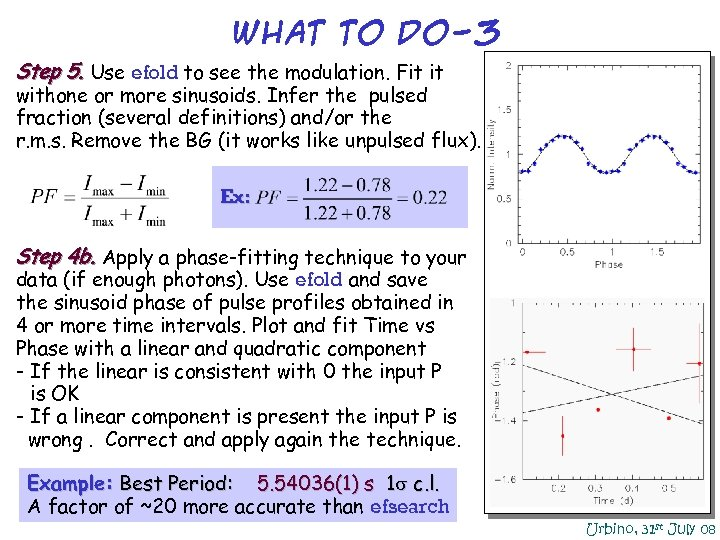What to do-3 Step 5. Use efold to see the modulation. Fit it withone or more sinusoids. Infer the pulsed fraction (several definitions) and/or the r. m. s. Remove the BG (it works like unpulsed flux). Ex: Step 4 b. Apply a phase-fitting technique to your data (if enough photons). Use efold and save the sinusoid phase of pulse profiles obtained in 4 or more time intervals. Plot and fit Time vs Phase with a linear and quadratic component - If the linear is consistent with 0 the input P - is OK - If a linear component is present the input P is wrong. Correct and apply again the technique. Example: Best Period: 5. 54036(1) s 1 c. l. A factor of ~20 more accurate than efsearch Urbino, 31 st July 08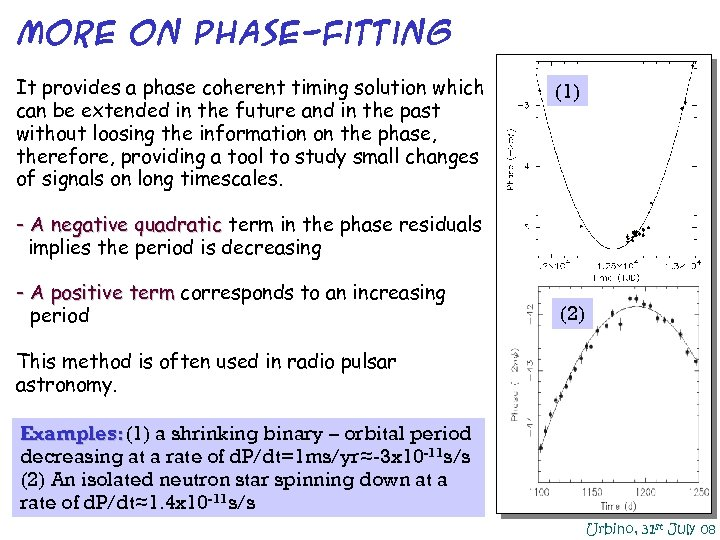MORE on phase-fitting It provides a phase coherent timing solution which can be extended in the future and in the past without loosing the information on the phase, therefore, providing a tool to study small changes of signals on long timescales. (1) - A negative quadratic term in the phase residuals implies the period is decreasing - A positive term corresponds to an increasing - period (2) This method is often used in radio pulsar astronomy. Examples: (1) a shrinking binary – orbital period decreasing at a rate of d. P/dt=1 ms/yr≈-3 x 10 -11 s/s (2) An isolated neutron star spinning down at a rate of d. P/dt≈1. 4 x 10 -11 s/s Urbino, 31 st July 08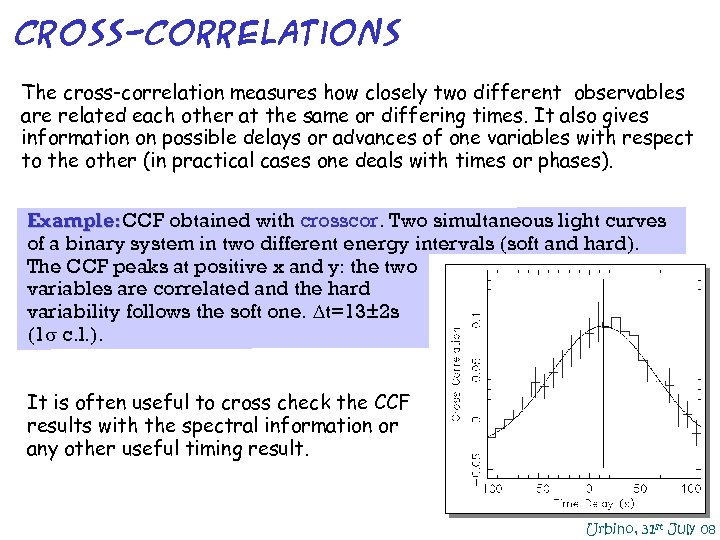Cross-correlations The cross-correlation measures how closely two different observables are related each other at the same or differing times. It also gives information on possible delays or advances of one variables with respect to the other (in practical cases one deals with times or phases). Example: CCF obtained with crosscor. Two simultaneous light curves of a binary system in two different energy intervals (soft and hard). The CCF peaks at positive x and y: the two variables are correlated and the hard variability follows the soft one. Dt=13± 2 s (1 c. l. ). It is often useful to cross check the CCF results with the spectral information or any other useful timing result. Urbino, 31 st July 08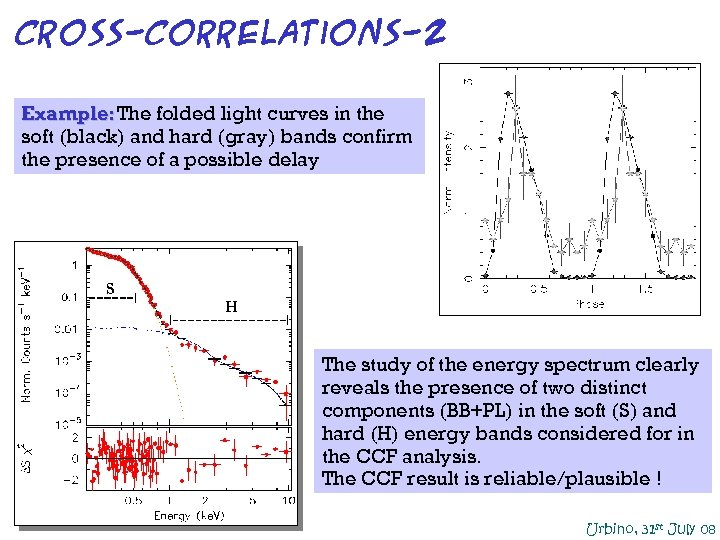Cross-correlations-2 Example: The folded light curves in the soft (black) and hard (gray) bands confirm the presence of a possible delay S H The study of the energy spectrum clearly reveals the presence of two distinct components (BB+PL) in the soft (S) and hard (H) energy bands considered for in the CCF analysis. The CCF result is reliable/plausible ! Urbino, 31 st July 08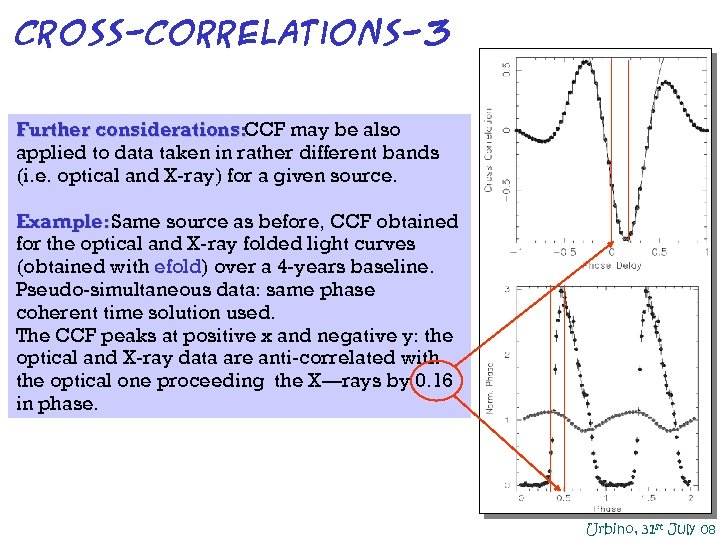Cross-correlations-3 Further considerations: CCF may be also applied to data taken in rather different bands (i. e. optical and X-ray) for a given source. Example: Same source as before, CCF obtained for the optical and X-ray folded light curves (obtained with efold) over a 4 -years baseline. Pseudo-simultaneous data: same phase coherent time solution used. The CCF peaks at positive x and negative y: the optical and X-ray data are anti-correlated with the optical one proceeding the X—rays by 0. 16 in phase. Urbino, 31 st July 08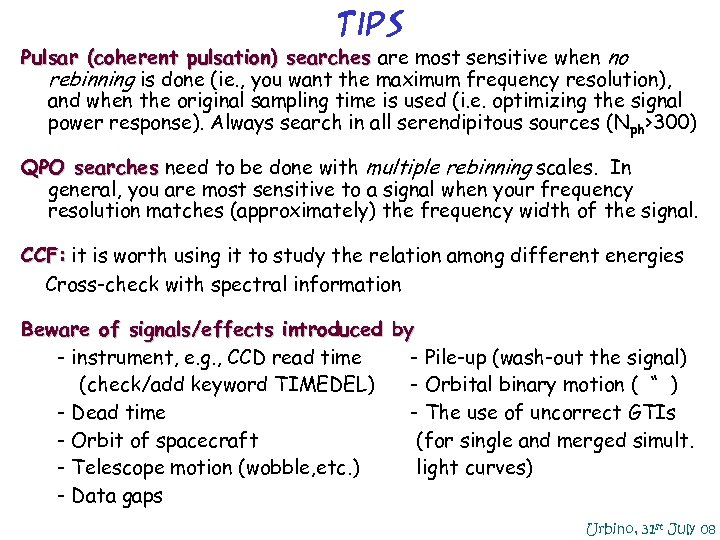Tips Pulsar (coherent pulsation) searches are most sensitive when no rebinning is done (ie. , you want the maximum frequency resolution), and when the original sampling time is used (i. e. optimizing the signal power response). Always search in all serendipitous sources (Nph>300) QPO searches need to be done with multiple rebinning scales. In general, you are most sensitive to a signal when your frequency resolution matches (approximately) the frequency width of the signal. CCF: it is worth using it to study the relation among different energies Cross-check with spectral information Beware of signals/effects introduced by - instrument, e. g. , CCD read time - Pile-up (wash-out the signal) - (check/add keyword TIMEDEL) - Orbital binary motion ( “ ) - Dead time - The use of uncorrect GTIs - Orbit of spacecraft (for single and merged simult. - Telescope motion (wobble, etc. ) light curves) - Data gaps Urbino, 31 st July 08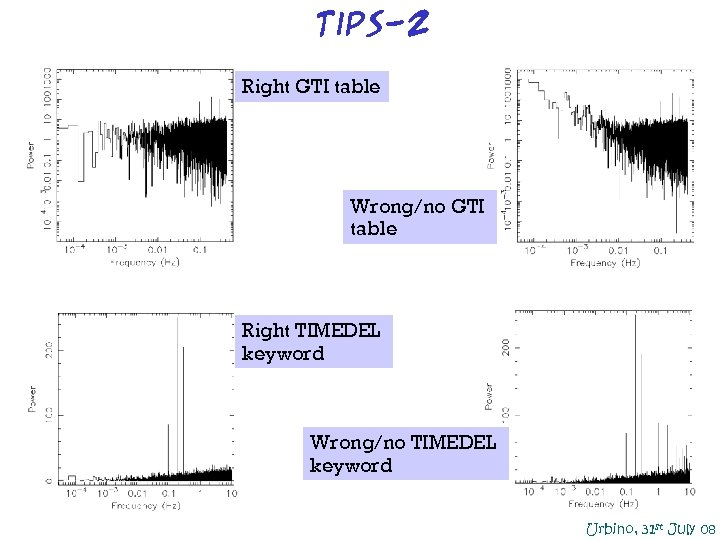Tips-2 Right GTI table Wrong/no GTI table Right TIMEDEL keyword Wrong/no TIMEDEL keyword Urbino, 31 st July 08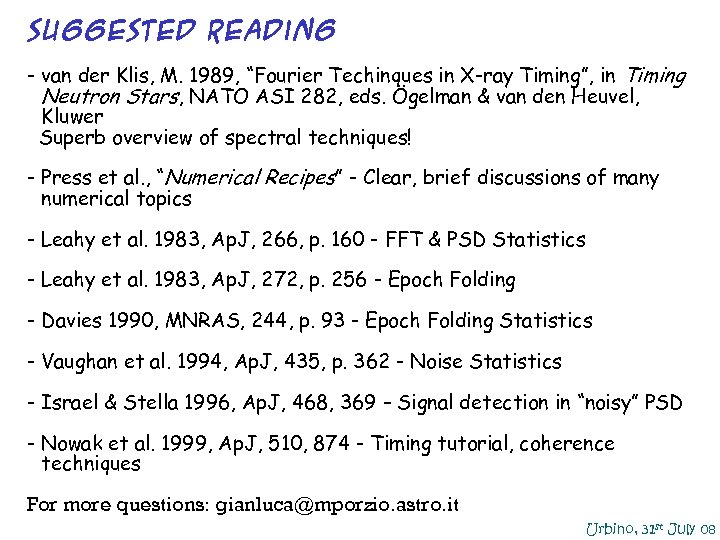Suggested Reading - van der Klis, M. 1989, “Fourier Techinques in X-ray Timing”, in Timing - Neutron Stars, NATO ASI 282, eds. Ögelman & van den Heuvel, - Kluwer Superb overview of spectral techniques! - Press et al. , “Numerical Recipes” - Clear, brief discussions of many - numerical topics - Leahy et al. 1983, Ap. J, 266, p. 160 - FFT & PSD Statistics - Leahy et al. 1983, Ap. J, 272, p. 256 - Epoch Folding - Davies 1990, MNRAS, 244, p. 93 - Epoch Folding Statistics - Vaughan et al. 1994, Ap. J, 435, p. 362 - Noise Statistics - Israel & Stella 1996, Ap. J, 468, 369 – Signal detection in “noisy” PSD - Nowak et al. 1999, Ap. J, 510, 874 - Timing tutorial, coherence - techniques For more questions: [email protected] astro. it Urbino, 31 st July 08Urbino, 31 st July 08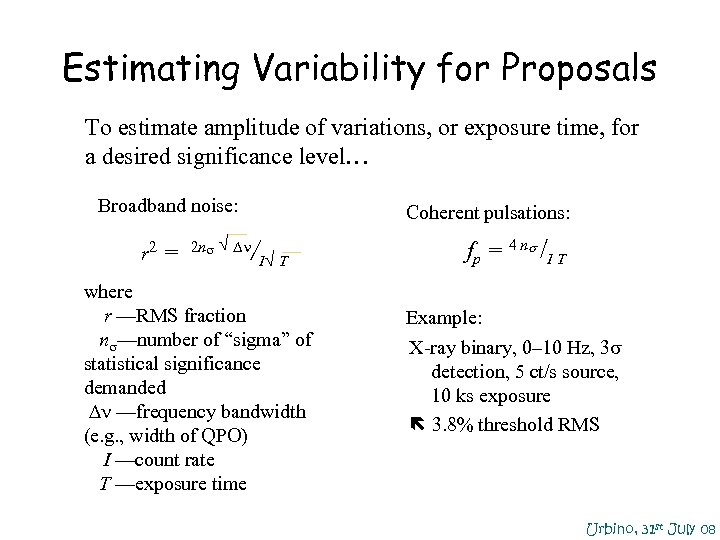Estimating Variability for Proposals To estimate amplitude of variations, or exposure time, for a desired significance level… • Broadband noise: r 2 = 2 n √ ∆ • Coherent pulsations: / I√ T where r —RMS fraction n —number of “sigma” of statistical significance demanded ∆ —frequency bandwidth (e. g. , width of QPO) I —count rate T —exposure time fp = 4 n /I T • Example: X-ray binary, 0– 10 Hz, 3 detection, 5 ct/s source, 10 ks exposure 3. 8% threshold RMS Urbino, 31 st July 08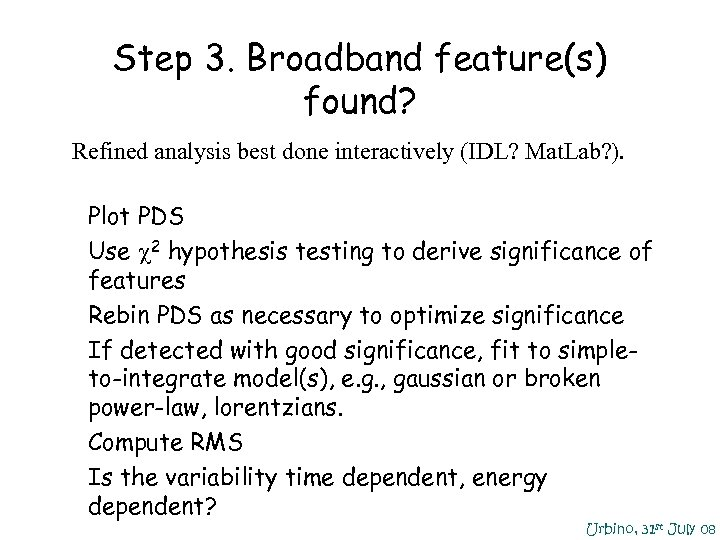Step 3. Broadband feature(s) found? Refined analysis best done interactively (IDL? Mat. Lab? ). • Plot PDS • Use 2 hypothesis testing to derive significance of features • Rebin PDS as necessary to optimize significance • If detected with good significance, fit to simpleto-integrate model(s), e. g. , gaussian or broken power-law, lorentzians. • Compute RMS • Is the variability time dependent, energy dependent? Urbino, 31 st July 08